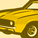# QlikView App Dev

Discussion Board for collaboration related to QlikView App Development.

cancel
Showing results for
Did you mean:Contributor III

## Top Analysis with multiple dimension in Pivot table

Hi Everyone ,

Good Morning. I am creating a Pivot Table with the help of below calculated dimensions.

=if(vSlider = 0,Product,if(Aggr(Rank(TOTAL Sum( Sales ) ), Product) <=vSlider,Product))

=if(vSlider = 0,Group,if(Aggr(Rank(TOTAL Sum( Sales ) ), Group) <= vSlider,Group))

And I am using the below expression

Sum({<Year={2017},Product=,Group=>}Sales)

I am using slider to get the top values from 5 to 30 separated by 5.

When i use a single dimension, the pivot table and Top analysis works fine and similarly when i use more than one dimension it doesn't works.

For Example , If i use only Product as a first dimension then it works and if i expand the pivot table with the second dimension, the top analysis doesn't works.

Suppress when value is null is checked.

Kindly help me on the above scenario.

Best Regards,

Siva

1 Solution

Accepted SolutionsSpecialist II

Aggr(Rank(TOTAL Sum( Sales ) ), Product, secondDimension)

i have not tested that. Eventually you Need build a condition

if(dimensionality()=1, aggr(..., Product),

if(dimensionality()=2, aggr(..., Product, SecondDimension),

2 RepliesSpecialist II

Aggr(Rank(TOTAL Sum( Sales ) ), Product, secondDimension)

i have not tested that. Eventually you Need build a condition

if(dimensionality()=1, aggr(..., Product),

if(dimensionality()=2, aggr(..., Product, SecondDimension),MVP

Would you be able to share a sample to look at the issue?Community Browser